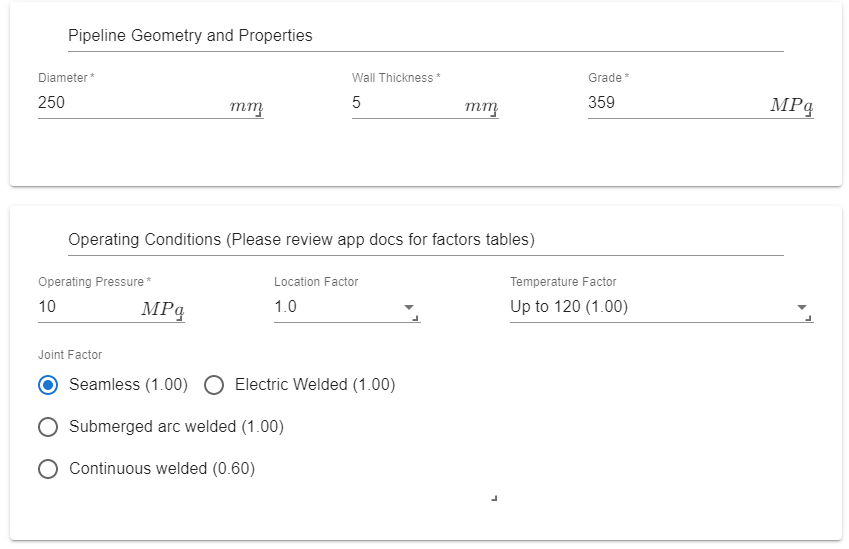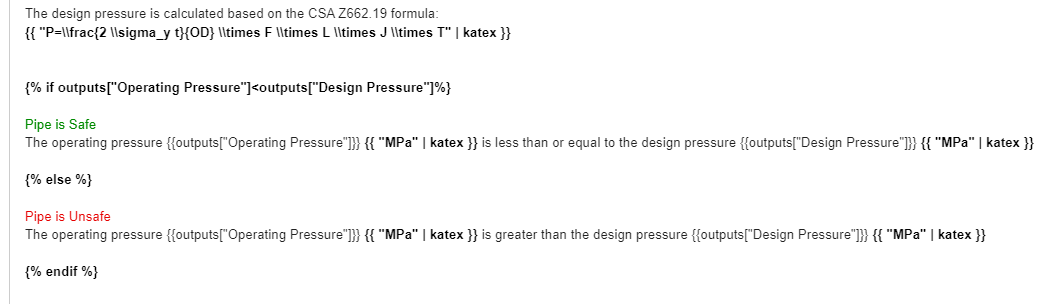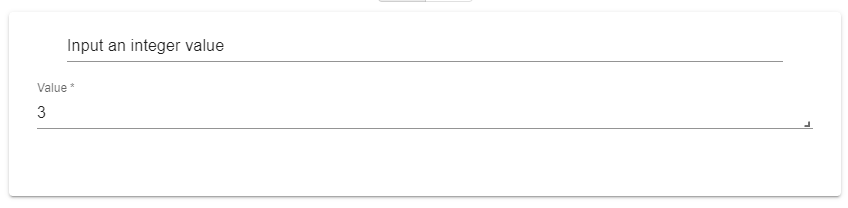# Conditionals

The Outputs editor is built upon Jinja, a templating engine for Python. Jinja operations can easily be identified with having {{ }} or {% %}. To learn more about templating visit the Jinja docs here or the Nunjucks docs here.

## If Statement​

{% if test1 %}# output if test1 is true{% elif test2 %}# output if test2 is true{% else test2 %}# output if all previous tests  are false{%  endif %}

### Example​

In this app, the CSA Z662.19 design equations are used to calculate the design pressure of a pipe. The app then compares the design pressure with the operating pressure and the if condition is used in the output to indicate whether the pipe is safe or unsafe.

Seven inputs are used:

• Four are Numerical Inputs: the Diameter d, the Wall Thickness t, the Grade sy, and the Operating Pressure p.
• Three are Single Select: the Location Factor l, the Temperature Factor tf, and the Joint Factor j.The code for this app is given below:

def main(inputs):    d = inputs['d']    t = inputs['t']    sy = inputs['sy']    p = inputs['p']    lf = inputs['l']    ldic = {"1.0":1.0, "0.9":0.90, "0.8":0.80, "0.75":0.75, "0.7":0.7, "0.625":0.625, "0.55":0.55, "0.5":0.5 }    ln = ldic[lf]    jf = inputs['j']    jdic = {"Seamless (1.00)":1.0, "Electric Welded (1.00)":1.0, "Submerged arc welded (1.00)":1.0, "Continuous welded (0.60)":0.6}    jn = jdic[jf]    tf = inputs['tf']    tdic = {"Up to 120 (1.00)": 1.0, "150 (0.97)":0.97, "180 (0.93)":0.93, "200 (0.91)":0.91, "230 (0.87)":0.87}    tn = tdic[tf]    dp = 2*sy*t/d*0.8*ln*jn*tn    dp = round(dp,2)    return {"Operating Pressure": p, "Design Pressure":dp}

Notice how the Single Select variables, which are strings are treated differently from the Numerical Inputs. A dictionary is used to find the factors corresponding to the options selected by the user. The code returns a dictionary with two keys: Operating Pressure and Design Pressure.

The if condition compares the two variables with each other to display a different message based on this comparison. The following is the text in the Output editor (Notice that the output variables were used in the if statement without the curly brackets):## For Statement​

{% for i in foo %}# output for every i{% endfor %}

### Example​

In this app, the user inputs an integer value. The app then outputs a table of all the numbers up to the integer value along with their squares:

The user is required to input an integer value a.The code simply outputs a dictionary with one key Count:

def main(inputs):    a = inputs['a']    return {"Count": a}

In the output editor, a condition along with the HTML table tags are used to generate a table based on the value of Count.

<table>  <th>Number</th>  <th>It's square</th>  {% for i in range(outputs.Count) %}  <tr>    <td>{{ i+1 }}</td>    <td>{{ (i+1)**2 }}</td>  </tr>  {% endfor %}</table>

Notice how the Python function range was used in the for loop and that inside the for loop, the output variable outputs.Count was used without any curly brackets. Notice as well how arithmetic operations (adding of the value of 1 and squaring the numbers) are done within two sets of curly brackets {{ }}.